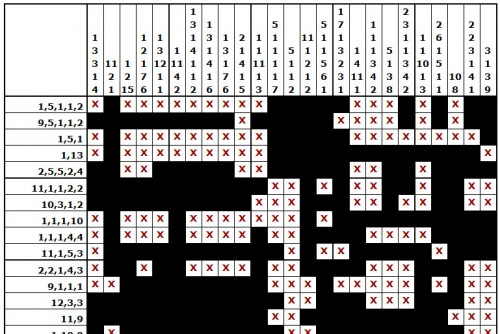# Printable Numbergrids### Printable Numbergrids

Numbergrid puzzles are a special kind of logic puzzle, where the goal is to uncover the pattern of black squares hidden within a grid. A series of numbers is provided for every row and column which defines that row or column’s sequence of black squares. “1,4,1”, for example, means that there is 1 black square, followed by an indeterminate number of spaces, followed by 4 consecutive black squares, followed by another indeterminate number of spaces, followed by a final single black square.

Each puzzle has one and only one unique solution, and each can be solved using only pure logical deduction – guessing and trial-and-error methods are never required for these puzzles.

Numbergrid Puzzles